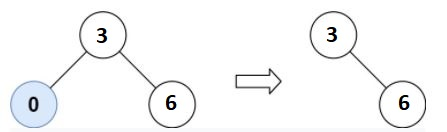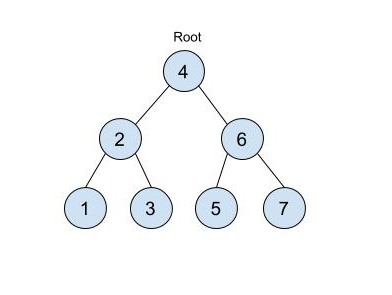# Modify BST

Posted: 12 Mar, 2021
Difficulty: Moderate

## PROBLEM STATEMENT

#### For example: For ‘LOW’ = 3 and ‘HIGH’ = 6 see the below picture for reference:``````As we can see 0 is not in the range [3, 6] so we delete this node from the ‘TREE’.
``````
##### Input Format:
``````The first line of input contains an integer 'T' representing the number of test cases. Then the test cases follow.

The first line of each test case contains two single space-separated integers ‘LOW’ and ‘HIGH’.

The second line of each test case contains elements in the level order form. The line consists of values of nodes separated by a single space. In case a node is null, we take -1 in its place.

For example, the input for the tree depicted in the below image would be :
````````````4
2 6
1 3 5 7
-1 -1 -1 -1 -1 -1
``````

#### Explanation :

``````Level 1 :
The root node of the tree is 4

Level 2 :
Left child of 4 = 2
Right child of 4 = 6

Level 3 :
Left child of 2 = 1
Right child of 2 = 3
Left child of 6 = 5
Right child of 6 = 7

Level 4 :
Left child of 1 = null (-1)
Right child of 1 = null (-1)
Left child of 3 = null (-1)
Right child of 3 = null (-1)
Left child of 5 = null (-1)
Right child of 5 = null (-1)
Left child of 7 = null (-1)
Right child of 7 = null (-1)

The first not-null node(of the previous level) is treated as the parent of the first two nodes of the current level. The second not-null node (of the previous level) is treated as the parent node for the next two nodes of the current level and so on.
The input ends when all nodes at the last level are null(-1).
``````
##### Note :
``````The above format was just to provide clarity on how the input is formed for a given tree.

The sequence will be put together in a single line separated by a single space. Hence, for the above-depicted tree, the input will be given as:

4 2 6 1 3 5 7 -1 -1 -1 -1 -1 -1
``````
##### Output Format :
``````For each test case, print the modified tree after trimming in the level order form.

The output for each test case is printed in a separate line.
``````
##### Note:
``````You do not need to print anything, it has already been taken care of. Just implement the given function.
``````
##### Constraints :
``````1 <= T <= 100
1 <= N <= 10 ^ 4
1 <= DATA <= 10 ^ 4
1 <= LOW , HIGH <= 10 ^ 4

Where N is the number of nodes in the ‘TREE’, ‘DATA’ represents the value of the node.

Time Limit: 1 Sec
``````Approach 1

The idea behind this approach is to use the following properties of BST:

• Values of the nodes in the left subtree of a node must be less than the value of the current node.
• Values of the nodes in the right subtree of a node must be greater than the value of the current node.

Now we do inorder traversal on ‘TREE’ and we need to handle the following cases:

• If the node value is in the range [‘LOW’, ‘HIGH’]:
• We need to return this node, but we may need to modify its left and right subtrees.
• Else if the node value is less than ’LOW’:
• Because of the binary search tree property, the value of the node as well as the value of all the nodes in the left subtree is not in range. So we need to return the modified right subtree.
• Else if the node value is greater than  ‘HIGH’:
• Because of the binary search tree property, the value of the node as well as the value of all the nodes in the right subtree is not in range. So we need to return the modified left subtree.

Here is the complete algorithm:

1. We call out recursive function ‘INORDERTRAVERSAL’ having parameters ‘CURRNODE’ representing the current node of the ‘TREE’ and ‘LOW’ and ‘HIGH’ respectively.
2. Initially, we pass the root as ‘CURRNODE’.
3. If the value of ‘CURRNODE’ is ‘NULL’, we simply return ‘NULL’.
4. If the value of the ‘CURRNODE’ is less than ‘LOW’.
• We call ‘INORDERTRAVERSAL’ function and pass ‘CURRNODE -> RIGHT’ as ‘CURRNODE’.
• Return the node returned by this call.
5. If the value of the ‘CURRNODE’ is greater than ‘HIGH’:
• We call ’INORDERTRAVERSAL’ function and pass ‘CURRNODE→LEFT’ as ‘CURRNODE’.
• Return the node returned by this call.
6. If the value of ‘CURRNODE’ is in range then do the following:
• Call  ‘INORDERTRAVERSAL’ and pass ‘CURRNODE -> LEFT’ as ‘CURRNODE’ and assign the modified left subtree to the left of ‘CURRNODE’.
• Call  ‘INORDERTRAVERSAL’ and pass ‘CURRNODE -> RIGHT’ as ‘CURRNODE’ and assign the modified right subtree to the right of ‘CURRNODE’.
7. Finally, return ‘CURRNODE’.